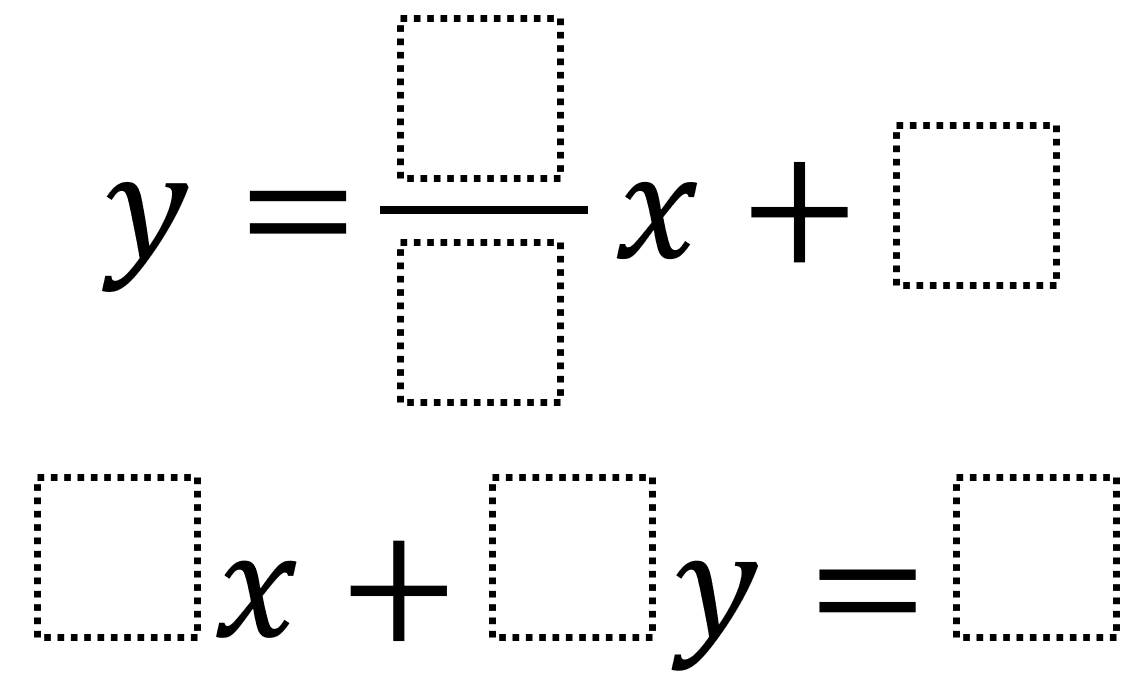# Perpendicular Lines 2

Directions: Using the digits 0 to 9 at most one time each, fill in the boxes to create two perpendicular lines whose solution is as close to the origin as possible.### Hint

How can you algebraically verify that your lines are perpendicular? How can you graphically verify that your lines are perpendicular?

The closest solution to the origin that’s been found so far comes from the lines y = (3/2)x + 0 and 4x + 6y = 1 which has a solution at (1/13, 3/26).

Source: Robert Kaplinsky

## Similar Triangles 2

Directions: Using the digits 0 to 9 at most one time each, create two similar …

### One comment

1.I believe y=3/2x + 0 and 6x + 9y = 1 gets a little bit closer